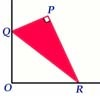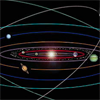#### You may also like### Set Square

A triangle PQR, right angled at P, slides on a horizontal floor with Q and R in contact with perpendicular walls. What is the locus of P?### Mach Attack

Have you got the Mach knack? Discover the mathematics behind exceeding the sound barrier.### Construct the Solar System

Make an accurate diagram of the solar system and explore the concept of a grand conjunction.

# Five Circuits, Seven Spins

### Why do this problem?

This is a good problem for discussion and developing clear visualisation and mathematical communication. It relates the angle of rotation of a circle to a distance and is therefore of use in exploring radians and the formula $s=r\theta$.

### Possible approach

Students' abilities to visualise the meaning of this problem might vary considerably. As such, this problem can appear to be difficult until a clear approach to the solution is found. The behaviour of the disc at the corners is likely to cause the most difficulty in imagining the rotation. As a result, students might need to be given a variety of visual devices to allow them to get started. For example:
• Imagine looking down onto the tray and watching the disc rotate about its centre.
• Imagine breaking the journey into a series of straight line trips.
• Imagine that the disc is pinned down in the centre and the tray is a track moved around the disc.
• Imagine that the edge of the disc is coated in ink. Which parts of the tray would be coloured following a lap of the track?
• Roll a coin around a book and use the head on the coin as a reference. Does the head rotate as it moves through a corner (i.e. when moving from a horizontal to a vertical part of the the journey).
This is the sort of problem which becomes much clearer once a solution has been found. Once students have solved the problem they should try to rewrite their answer and method as clearly as possible, in a way which is both simple but complete.

It is possible to tackle this problem using degrees and the formula for the circumference of a circle, but it is much simpler to solve using radians and the formula $s=r\theta$.

### Key questions

How far does the centre of a disc move in one revolution when the plate is in contact with a straight edge?

What mathematics allows us to relate this distance to an angle?

What units should we measure the angle of rotation in? Why?

If the disc has rotated $7$ full times, how far must it have rolled?

As the disc makes a single lap of the tray, what parts of the tray will have made contact with the disc? How far is this?

### Possible support

Consider the distance a bicycle travels when the wheels rotate once.

Read the article A Rolling Disc - Periodic Motion.

### Possible extension

Try the problem Contact